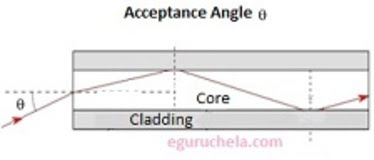### Acceptance Angle in Optical Fibre Calculation

The system calculate the acceptance angle of an optical fiber using following formula:
Formula: θm = sin-1((1/ηo) * (ηf2c2)1/2)
Where, θm = Acceptance Angle, ηo = Exterior Refractive Index, ηf = Fibre Refractive Index, ηc = Cladding Refractive IndexExterior Refractive Index: Fibre Refractive Index: Cladding Refractive Index: Acceptance Angle: °
The acceptance angle is the maximum angle at which light-ray enters or hits the fiber core and getting propagate though it in zig-zag path. The acceptable angle is also called the numerical aperture therefore it is determined by the refractive index contrast between core and cladding of the fiber with assumption that the incident beam comes from air or vacuum.